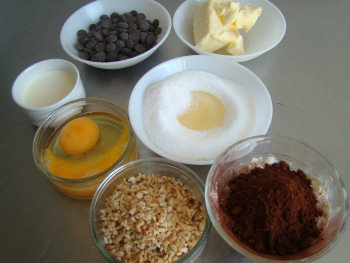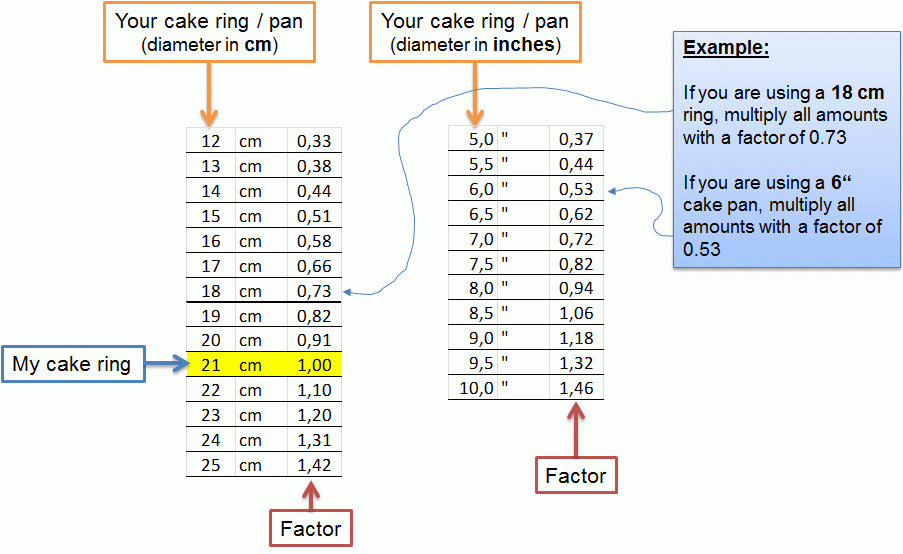# LESSON: Measuring Ingredients

1. Measuring dry ingredients
2. Measuring eggs
3. Measuring liquids
4. Different pan sizes
5. Conversions

## Baking is a ScienceThe members of keikos-cake come from all over the world. We have pastry friends from the US, UK, Malaysia, Singapore, Australia… and many other countries. When I list the ingredients for a recipe, I must be sure that members in all countries will use the same amounts of ingredients as I do here in Germany.

With “same amounts”, I mean the same proportions of weight.

Baking is a combination of chemistry and physics. It’s a lot like science. Baking a cake gives the same results when the same ingredients and amounts are used, under the same conditions, following the same steps.

That’s great because it enables me to show you how to make perfect cakes, no matter where you live. 🙂
There’s no guesswork.

And it all starts with measuring ingredients. I list the amounts in my recipes in units of weight: grams and ounces. In some countries, ingredients are also measured in units of volume like ‘cups’ or ‘liter’. Let’s see if that’s a good idea…

## 1. Measuring Dry Ingredients

I sometimes get emails from pastry fans who ask me to provide the amounts in ‘US measurements’ (meaning ‘cups’ and ‘eggs’).

‘Cups’ measure the volume of an ingredient. In baking, it’s important to use the same weights to get the same results.

Now, that would be no problem as long as the following is true (I choose sugar as an example):

‘One cup of sugar always weighs the same.’ — True? (yes/no)

Is that true? What do you think? Let’s check… (watch the video):

How is it possible that one cup (or glass) of sugar weighs 17% less than another cup of sugar?

The reason here is the slightly different grain size. Each brand of sugar has a different grain size, so a ‘cup of sugar’ is different for each brand. That means, if I would list the amounts in my recipes in ‘cups’, you would only get the correct proportions if you use the exact same brands that I use. That’s not practicable with pastry friends living in all parts of the world…

The solution: Always measure dry ingredients in units of weight (grams, ounces).

That’s the reason why a scale is the second most important tool for a serious baker (the most important tool is the oven). A scale is cheap and solves all problems related to measuring ingredients. This is the scale that I use in the video.

## 2. Measuring Eggs

Eggs come in many different sizes. HERE is a short list of “egg sizes” in different countries.

According to that list, an egg that weighs 62 grams is called:

• “Medium” in Europe
• “Large” in USA and Canada
• “Extra-Large” in Australia

… quite confusing. 🙂

And even within one weight range, egg weights can differ 16% and more. For example, a European “medium” egg can weigh anywhere from 53g to 62g.

#### Can you make a perfect souffle using 16% too much (or too few) egg white?

No, that’s impossible. Making a perfect souffle (and many other treats) becomes a game of pure chance if you only count the number of eggs.

The solution: Always measure eggs in units of weight!

The video shows how to do it correctly…

## 3. Measuring liquids

Liquids can be measured in units of volume (ml) or weight (g, oz).
For liquids, it’s usually true that same volumes of liquid have the same weight.

In my recipes, I mostly measure the liquid ingredients in units of weight (g, oz.) and not volume (ml). It’s just a lot easier to measure for example 127 g water with a scale than it is to measure 127 ml of water with a measuring cup. 🙂

However, you can always just exchange grams with ml. For example, if a recipe asks for 100 g water, you can measure 100 ml of water with a measuring cup, instead. This also works for milk and heavy cream. They have a slightly different density than water, but the difference is so small that it doesn’t matter (example: heavy cream with 35% fat has a density of 1.004 kg/l which is practically the same as water).

## 4. Different pan size

When you use a different pan size than I do in the cake guide, you will need to adapt the amounts of all ingredients. If your cake pan is bigger, you will need more ingredients. If your pan is smaller, you can use less.

### Round Cakes

Below are the conversion factors for typical cake diameters that I use in my recipes.

Original recipe: 18 cm diameterOriginal recipe: 21 cm diameterWhat if your pan size is not listed above? Use the general formula…

The general formula is: conversion factor = yourRingArea / myRingArea
Tip: Area of a circle = PI*radius^2 😀

Here’s an example:
The original center ring has a diameter of 14 cm –> radius = 7 cm (2.76 inches)
Your ring has a diameter of 6 inches (15.24 cm) –> radius = 7.62 cm (3 inches)

conversion factor = (PI * 3^2) / (PI * 2.76^2) = 3^2 / 2.76^2 = 9 / 7.6176 = 1.2 (rounded)

### Rectangular Cakes

For rectangular shapes like the sponge cake sheet (Swiss roll), follow these steps to calculate the conversion factor:

1. Calculate the area of the pan in the original recipe. (Area = sideA*sideB)
2. Calculate the area of your pan.
3. Divide both to get the conversion factor, i.e. factor = Area(your pan) / Area(original recipe)
4. IMPORTANT: Use the same units (cm, inches) when calculating the two areas.

## 5. Conversions

It should be clear by now that the serious baker measures ingredients with a scale. However, if you need to convert amounts from cups to grams or vice versa, you need a good conversion tool. The best one that I’ve found online is THIS. With this tool, you could convert my recipes into ‘cups’, for example. As explained above, I do NOT recommend doing this, as it turns the science of baking into a game of pure chance.

More lessons coming soon… 🙂